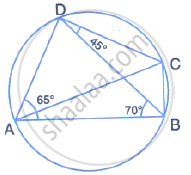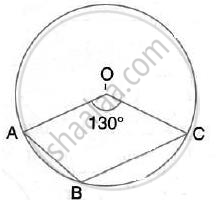Share

In the Figure, ∠Bad = 65° , ∠Abd = 70° , ∠Bdc = 45° (I) Prove that Ac is a Diameter of the Circle (Ii) Find ∠Acb - ICSE Class 10 - Mathematics

ConceptArc and Chord Properties - Angles in the Same Segment of a Circle Are Equal (Without Proof)

Question

In the figure, ∠BAD  = 65° , ∠ABD = 70° , ∠BDC = 45°
(i) Prove that AC is a diameter of the circle
(ii) Find ∠ACBSolutionHere, Reflex  ∠AOC= 2∠ABC
(Angle at the centre is double the angle at the circumference subtended by the same chord)
⇒  360° - 130° =2∠ABC
⇒   ∠ABC = (230°)/2 = 115°

Is there an error in this question or solution?
Solution In the Figure, ∠Bad = 65° , ∠Abd = 70° , ∠Bdc = 45° (I) Prove that Ac is a Diameter of the Circle (Ii) Find ∠Acb Concept: Arc and Chord Properties - Angles in the Same Segment of a Circle Are Equal (Without Proof).
S